xEnthalpyEncyclopedia
Enthalpy is a measure of the total energy
Energy
In physics, energy is an indirectly observed quantity. It is often understood as the ability a physical system has to do work on other physical systems...

of a thermodynamic system
Thermodynamic system
A thermodynamic system is a precisely defined macroscopic region of the universe, often called a physical system, that is studied using the principles of thermodynamics....

. It includes the internal energy
Internal energy
In thermodynamics, the internal energy is the total energy contained by a thermodynamic system. It is the energy needed to create the system, but excludes the energy to displace the system's surroundings, any energy associated with a move as a whole, or due to external force fields. Internal...

, which is the energy required to create a system, and the amount of energy required to make room for it by displacing its environment
Environment (systems)
In science and engineering, a system is the part of the universe that is being studied, while the environment is the remainder of the universe that lies outside the boundaries of the system. It is also known as the surroundings, and in thermodynamics, as the reservoir...

and establishing its volume and pressure.

Enthalpy is a thermodynamic potential. It is a state function
State function
In thermodynamics, a state function, function of state, state quantity, or state variable is a property of a system that depends only on the current state of the system, not on the way in which the system acquired that state . A state function describes the equilibrium state of a system...

and an extensive quantity. The unit of measurement for enthalpy in the International System of Units
International System of Units
The International System of Units is the modern form of the metric system and is generally a system of units of measurement devised around seven base units and the convenience of the number ten. The older metric system included several groups of units...

(SI) is the joule
Joule
The joule ; symbol J) is a derived unit of energy or work in the International System of Units. It is equal to the energy expended in applying a force of one newton through a distance of one metre , or in passing an electric current of one ampere through a resistance of one ohm for one second...

, but other historical, conventional units are still in use, such as the British thermal unit
British thermal unit
The British thermal unit is a traditional unit of energy equal to about 1055 joules. It is approximately the amount of energy needed to heat of water, which is exactly one tenth of a UK gallon or about 0.1198 US gallons, from 39°F to 40°F...

and the calorie
Calorie
The calorie is a pre-SI metric unit of energy. It was first defined by Nicolas Clément in 1824 as a unit of heat, entering French and English dictionaries between 1841 and 1867. In most fields its use is archaic, having been replaced by the SI unit of energy, the joule...

.

The enthalpy is the preferred expression of system energy changes in many chemical, biological, and physical measurements, because it simplifies certain descriptions of energy transfer
Energy transfer
Energy transfer is the transfer of energy from one body to another.There are a few main ways that energy transfer occurs:*Radiant energy *Heat conduction*Convection*Electrical power transmission*Mechanical work...

. This is because a change in enthalpy takes account of energy transferred to the environment through the expansion of the system under study.

The total enthalpy, H, of a system cannot be measured directly. Thus, change in enthalpy, ΔH, is a more useful quantity than its absolute value. The change ΔH is positive in endothermic
Endothermic
In thermodynamics, the word endothermic describes a process or reaction in which the system absorbs energy from the surroundings in the form of heat. Its etymology stems from the prefix endo- and the Greek word thermasi,...

reactions, and negative in exothermic
Exothermic
In thermodynamics, the term exothermic describes a process or reaction that releases energy from the system, usually in the form of heat, but also in the form of light , electricity , or sound...

processes. ΔH of a system is equal to the sum of non-mechanical work done on it and the heat supplied to it.

For quasistatic processes under constant pressure, ΔH is equal to the change in the internal energy of the system, plus the work that the system has done on its surroundings. This means that the change in enthalpy under such conditions is the heat absorbed (or released) by a chemical reaction.

## Formal definition

H(S,p), can be derived as a thermodynamic potential naturally dependent on S and p can be derived as follows from U(S,V). Here, U is internal energy, T is absolute temperature, S is entropy, p is pressure, and V is volume:
The fundamental thermodynamic relation is basically the First Law of thermodynamics for reversible processes: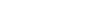Apply the product for differentiation to pV: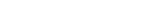, hence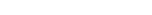The enthalpy of a system is defined as: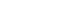so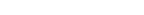where
H is the enthalpy of the system
U is the internal energy
Internal energy
In thermodynamics, the internal energy is the total energy contained by a thermodynamic system. It is the energy needed to create the system, but excludes the energy to displace the system's surroundings, any energy associated with a move as a whole, or due to external force fields. Internal...

of the system
p is the pressure
Pressure
Pressure is the force per unit area applied in a direction perpendicular to the surface of an object. Gauge pressure is the pressure relative to the local atmospheric or ambient pressure.- Definition :...

at the boundary of the system and its environment
V is the volume of the system.

Note that the U term is equivalent to the energy required to create the system, and that the pV term is equivalent to the energy that would be required to "make room" for the system if the pressure of the environment remained constant.

The pV term may be understood by the following example of an isobaric process
Isobaric process
An isobaric process is a thermodynamic process in which the pressure stays constant. The term derives from the Greek isos, , and barus,...

. Consider gas changing its volume (by, for example, a chemical reaction) in a cylinder, pushing a piston, maintaining constant pressure p. The force is calculated from the area A of the piston and definition of pressure p = F/A: the force is F = pA. By definition, work W done is W = Fx, where x is the distance traversed. Combining gives W = pAx, and the product Ax is the volume traversed by the piston: Ax = V. Thus, the work done by the gas is W = pV, where p is a constant pressure and V the expansion of volume. Including this pV term means that during constant pressure expansion, any internal energy forfeited as work on the environment does not affect the value of enthalpy. The enthalpy change can be defined ΔH = ΔU + W = ΔU + Δ(pV), where ΔU is the thermal energy lost to expansion, and W the energy gained due to work done on the piston.

### Difference between enthalpy and internal energy

Chemists routinely use H as the energy of the system, but the pV term is not stored in the system, but rather in the surroundings, such as the atmosphere. When a system, for example, n moles
Mole (unit)
The mole is a unit of measurement used in chemistry to express amounts of a chemical substance, defined as an amount of a substance that contains as many elementary entities as there are atoms in 12 grams of pure carbon-12 , the isotope of carbon with atomic weight 12. This corresponds to a value...

of a gas of volume V at pressure
Pressure
Pressure is the force per unit area applied in a direction perpendicular to the surface of an object. Gauge pressure is the pressure relative to the local atmospheric or ambient pressure.- Definition :...

P and temperature
Temperature
Temperature is a physical property of matter that quantitatively expresses the common notions of hot and cold. Objects of low temperature are cold, while various degrees of higher temperatures are referred to as warm or hot...

T, is created or brought to its present state from absolute zero
Absolute zero
Absolute zero is the theoretical temperature at which entropy reaches its minimum value. The laws of thermodynamics state that absolute zero cannot be reached using only thermodynamic means....

, energy must be supplied equal to its internal energy U plus pV, where pV is the work done in pushing against the ambient (atmospheric) pressure. This additional energy is, therefore, stored in the surroundings and can be recovered when the system collapses back to its initial state. In basic chemistry
Chemistry
Chemistry is the science of matter, especially its chemical reactions, but also its composition, structure and properties. Chemistry is concerned with atoms and their interactions with other atoms, and particularly with the properties of chemical bonds....

scientists are typically interested in experiments conducted at atmospheric pressure
Atmospheric pressure
Atmospheric pressure is the force per unit area exerted into a surface by the weight of air above that surface in the atmosphere of Earth . In most circumstances atmospheric pressure is closely approximated by the hydrostatic pressure caused by the weight of air above the measurement point...

, and for reaction energy calculations they care about the total energy in such conditions, and therefore typically need to use H. In basic physics
Physics
Physics is a natural science that involves the study of matter and its motion through spacetime, along with related concepts such as energy and force. More broadly, it is the general analysis of nature, conducted in order to understand how the universe behaves.Physics is one of the oldest academic...

and thermodynamics
Thermodynamics
Thermodynamics is a physical science that studies the effects on material bodies, and on radiation in regions of space, of transfer of heat and of work done on or by the bodies or radiation...

it may be more interesting to study the internal properties of the system and therefore the internal energy is used.

## Origins

The word enthalpy is based on the Greek word enthalpos (ἔνθαλπος), which means to put heat into. It comes from the Classical Greek prefix ἐν-, en-, meaning to put into, and the verb θάλπειν, thalpein, meaning "to heat". The word enthalpy is often incorrectly attributed to Benoit Paul Émile Clapeyron
Benoit Paul Émile Clapeyron
Benoît Paul Émile Clapeyron was a French engineer and physicist, one of the founders of thermodynamics.-Life:...

and Rudolf Clausius
Rudolf Clausius
Rudolf Julius Emanuel Clausius , was a German physicist and mathematician and is considered one of the central founders of the science of thermodynamics. By his restatement of Sadi Carnot's principle known as the Carnot cycle, he put the theory of heat on a truer and sounder basis...

through the 1850 publication of their Clausius-Clapeyron relation
Clausius-Clapeyron relation
The Clausius–Clapeyron relation, named after Rudolf Clausius and Benoît Paul Émile Clapeyron, who defined it sometime after 1834, is a way of characterizing a discontinuous phase transition between two phases of matter. On a pressure–temperature diagram, the line separating the two phases is known...

. This misconception was popularized by the 1927 publication of The Mollier Steam Tables and Diagrams. However, neither the concept, the word, nor the symbol for enthalpy existed until well after Clapeyron's death.

The earliest writings to contain the concept of enthalpy did not occur until 1875,
when Josiah Willard Gibbs
Josiah Willard Gibbs
Josiah Willard Gibbs was an American theoretical physicist, chemist, and mathematician. He devised much of the theoretical foundation for chemical thermodynamics as well as physical chemistry. As a mathematician, he invented vector analysis . Yale University awarded Gibbs the first American Ph.D...

introduced "a heat function for constant pressure". However, Gibbs did not use the word "enthalpy" in his writings.The Collected Works of J. Willard Gibbs, Vol. I do not contain reference to the word enthalpy, but rather reference the heat function for constant pressure.
Instead, the word "enthalpy" first appears in the scientific literature in a 1909 publication by J. P. Dalton. According to that publication, the word was actually coined by Heike Kamerlingh Onnes
Heike Kamerlingh Onnes
Heike Kamerlingh Onnes was a Dutch physicist and Nobel laureate. He pioneered refrigeration techniques, and he explored how materials behaved when cooled to nearly absolute zero. He was the first to liquify helium...

.
Over the years, many different symbols were used to denote enthalpy. It was not until 1922 that Alfred W. Porter proposed the symbol "H" as the accepted standard, thus finalizing the terminology still in use today.

## Relationship to heat

The increase in enthalpy of a system is exactly equal to the energy added through heat
Heat
In physics and thermodynamics, heat is energy transferred from one body, region, or thermodynamic system to another due to thermal contact or thermal radiation when the systems are at different temperatures. It is often described as one of the fundamental processes of energy transfer between...

, provided that the system is under constant pressure and that the only work done on the system is expansion work: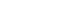where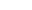is the change in enthalpy of the system (under the restrictions mentioned above), and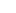is the energy added to the system through heat
Heat
In physics and thermodynamics, heat is energy transferred from one body, region, or thermodynamic system to another due to thermal contact or thermal radiation when the systems are at different temperatures. It is often described as one of the fundamental processes of energy transfer between...

.
Expansion work is the transfer of energy between the system and its environment through changes in the system's volume. This type of work does not affect the above equation. Any other type of work that could be done on the system aside from expansion is called non-mechanical work.
Non-mechanical work could include such processes as altering the internal energy using an external electric field
Electric field
In physics, an electric field surrounds electrically charged particles and time-varying magnetic fields. The electric field depicts the force exerted on other electrically charged objects by the electrically charged particle the field is surrounding...

, or adding energy through stirring. If any non-mechanical work takes place then the above equation will not hold.

The exact relationship between enthalpy and heat can be derived from the definition of enthalpy.

According to the definition of enthalpy,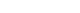where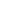is the enthalpy of the system,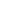is the internal energy
Internal energy
In thermodynamics, the internal energy is the total energy contained by a thermodynamic system. It is the energy needed to create the system, but excludes the energy to displace the system's surroundings, any energy associated with a move as a whole, or due to external force fields. Internal...

of the system,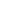is the pressure
Pressure
Pressure is the force per unit area applied in a direction perpendicular to the surface of an object. Gauge pressure is the pressure relative to the local atmospheric or ambient pressure.- Definition :...

at the boundary of the system and its environment, and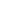is the volume
Volume
Volume is the quantity of three-dimensional space enclosed by some closed boundary, for example, the space that a substance or shape occupies or contains....

of the system.

Differentiating yields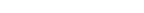According to the first law of thermodynamics
First law of thermodynamics
The first law of thermodynamics is an expression of the principle of conservation of work.The law states that energy can be transformed, i.e. changed from one form to another, but cannot be created nor destroyed...

, any changes in internal energy are due to energy transferred with the environment (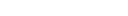), so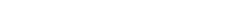where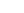is the infinitesimal amount of energy added to the system through heat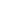is the infinitesimal amount of energy added to the system through expansion work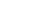is the infinitesimal amount of energy added to the system through any means other than heat or expansion work. (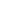is sometimes called non-mechanical work.)

Because the energy added to the system through expansion work is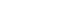, this term can cancel with the existing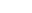term to yield: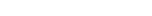integrating then yields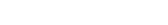Notice that this equation still has some extra terms; this is where the restrictions come in. Restricting the conditions to constant pressure ensures that the final term will equal zero. Ensuring that no work is done aside from expansion work makes the W' term zero. Thus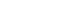Provided that the pressure is constant and that the only work done on the system is through system expansion.

### Enthalpy is not heat

Enthalpy is sometimes described as the heat content of a system under a given pressure, whereas "heat" is defined as thermal energy in transit. For the assumption that a change of enthalpyis heat to be valid, no energy exchange with the environment must occur aside from heat or expansion work. Given this restriction, it can be shown that:
• The enthalpy is the total amount of energy that the system can emit through heat
• Adding or removing energy through heat is one of two only ways to change the enthalpy
• The amount of change in enthalpy is equal to the amount of energy added through heat.

Thus it is as if enthalpy is nothing more than heat "stored" by the system, provided the given restrictions are adhered to.

However, heat is not the only way to change enthalpy. Enthalpy also changes when the pressure of the environment is altered, even if no energy is exchanged as heat. In addition, enthalpy changes when energy is transferred into or out of the system through a means other than heat or expansion work, such as through external fields or stirring.

## Applications

In thermodynamics, one can calculate enthalpy by determining the requirements for creating a system from "nothingness"; the mechanical work required, pV, differs based upon the constancy of conditions present at the creation of the thermodynamic system
Thermodynamic system
A thermodynamic system is a precisely defined macroscopic region of the universe, often called a physical system, that is studied using the principles of thermodynamics....

.

Internal energy
Internal energy
In thermodynamics, the internal energy is the total energy contained by a thermodynamic system. It is the energy needed to create the system, but excludes the energy to displace the system's surroundings, any energy associated with a move as a whole, or due to external force fields. Internal...

, U, must be supplied to remove particles from a surrounding in order to allow space for the creation of a system, providing that environmental variables, such as pressure (p) remain constant. This internal energy also includes the energy required for activation
Activation energy
In chemistry, activation energy is a term introduced in 1889 by the Swedish scientist Svante Arrhenius that is defined as the energy that must be overcome in order for a chemical reaction to occur. Activation energy may also be defined as the minimum energy required to start a chemical reaction...

and the breaking of bonded compounds into gaseous species.

This process is calculated within enthalpy calculations as U + pV, to label the amount of energy or work required to "set aside space for" and "create" the system; describing the work done by both the reaction or formation of systems, and the surroundings. For systems at constant pressure, the change in enthalpy is the heat received by the system.

Therefore, the change in enthalpy can be devised or represented without the need for compressive or expansive mechanics; for a simple system, with a constant number of particles, the difference in enthalpy is the maximum amount of thermal energy derivable from a thermodynamic process in which the pressure is held constant.

The term pV is the work required to displace the surrounding atmosphere in order to vacate the space to be occupied by the system.

### Heat of reaction

The total enthalpy of a system cannot be measured directly; the enthalpy change of a system is measured instead. Enthalpy change is defined by the following equation:
ΔH = Hfinal − Hinitial

where
ΔH  is the enthalpy change

Hfinal is the final enthalpy of the system, expressed in joules. In a chemical reaction, Hfinal is the enthalpy of the products.

Hinitial is the initial enthalpy of the system, expressed in joules. In a chemical reaction, Hinitial is the enthalpy of the reactants.

For an exothermic
Exothermic reaction
An exothermic reaction is a chemical reaction that releases energy in the form of light or heat. It is the opposite of an endothermic reaction. Expressed in a chemical equation:-Overview:...

reaction
Chemical reaction
A chemical reaction is a process that leads to the transformation of one set of chemical substances to another. Chemical reactions can be either spontaneous, requiring no input of energy, or non-spontaneous, typically following the input of some type of energy, such as heat, light or electricity...

at constant pressure
Pressure
Pressure is the force per unit area applied in a direction perpendicular to the surface of an object. Gauge pressure is the pressure relative to the local atmospheric or ambient pressure.- Definition :...

, the system's change in enthalpy equals the energy released in the reaction, including the energy retained in the system and lost through expansion against its surroundings. In a similar manner, for an endothermic
Endothermic
In thermodynamics, the word endothermic describes a process or reaction in which the system absorbs energy from the surroundings in the form of heat. Its etymology stems from the prefix endo- and the Greek word thermasi,...

reaction, the system's change in enthalpy is equal to the energy absorbed in the reaction, including the energy lost by the system and gained from compression from its surroundings. A relatively easy way to determine whether or not a reaction is exothermic or endothermic is to determine the sign of ΔH. If ΔH is positive, the reaction is endothermic, that is heat is absorbed by the system due to the products of the reaction having a greater enthalpy than the reactants. On the other hand if ΔH is negative, the reaction is exothermic, that is the overall decrease in enthalpy is achieved by the generation of heat.

Although enthalpy is commonly used in engineering and science, it is impossible to measure directly, as enthalpy has no datum (reference point). Therefore enthalpy can only accurately be used in a closed system
Closed system
-In physics:In thermodynamics, a closed system can exchange energy , but not matter, with its surroundings.In contrast, an isolated system cannot exchange any of heat, work, or matter with the surroundings, while an open system can exchange all of heat, work and matter.For a simple system, with...

. However, few real-world applications exist in closed isolation, and it is for this reason that two or more closed systems cannot be compared using enthalpy as a basis, although sometimes this is done erroneously.

### Specific enthalpy

The specific enthalpy of a working mass
Working mass
Working mass is a mass against which a system operates in order to produce acceleration.In the case of a rocket, for example, the reaction mass is the fuel shot backwards to provide propulsion. All acceleration requires an exchange of momentum, which can be thought of as the "unit of movement"...

is a property of that mass used in thermodynamics
Thermodynamics
Thermodynamics is a physical science that studies the effects on material bodies, and on radiation in regions of space, of transfer of heat and of work done on or by the bodies or radiation...

. It is defined as h = u + pv, where u is the specific internal energy
Internal energy
In thermodynamics, the internal energy is the total energy contained by a thermodynamic system. It is the energy needed to create the system, but excludes the energy to displace the system's surroundings, any energy associated with a move as a whole, or due to external force fields. Internal...

, p is the pressure, and v is specific volume. In other words, h = H/m where m is the mass of the system. The SI unit for specific enthalpy is joules per kilogram.

### Enthalpy changes

An enthalpy change describes the change in enthalpy observed in the constituents of a thermodynamic system when undergoing a transformation or chemical reaction. It is the difference between the enthalpy after the process has completed, i.e. the enthalpy of the products
Product (chemistry)
Product are formed during chemical reactions as reagents are consumed. Products have lower energy than the reagents and are produced during the reaction according to the second law of thermodynamics. The released energy comes from changes in chemical bonds between atoms in reagent molecules and...

, and the initial enthalpy of the system, i.e. the reactants. These processes are reversible and the enthalpy for the reverse process is the negative value of the forward change.

A common standard enthalpy change is the enthalpy of formation, which has been determined for a large number of substances. Enthalpy changes are routinely measured and compiled in chemical and physical reference works, such as the CRC Handbook of Chemistry and Physics
CRC Handbook of Chemistry and Physics
The CRC Handbook of Chemistry and Physics is in its 92nd edition . It is sometimes nicknamed the 'Rubber Bible' or the 'Rubber Book', as CRC originally stood for "Chemical Rubber Company"....

. The following is a selection of enthalpy changes commonly recognized in thermodynamics.

When used in these recognized terms the qualifier change is usually dropped and the property is simply termed enthalpy of 'process. Since these properties are often used as reference values it is very common to quote them for a standardized set of environmental parameters, or standard conditions, which is typically a temperature of and a pressure of either or . For such standardized values the name of the enthalpy is commonly prefixed with the term standard, e.g. standard enthalpy of formation.

Chemical properties:
• Enthalpy of reaction, defined as the enthalpy change observed in a constituent of a thermodynamic system when one mole
Mole (unit)
The mole is a unit of measurement used in chemistry to express amounts of a chemical substance, defined as an amount of a substance that contains as many elementary entities as there are atoms in 12 grams of pure carbon-12 , the isotope of carbon with atomic weight 12. This corresponds to a value...

of substance reacts completely.
• Enthalpy of formation, defined as the enthalpy change observed in a constituent of a thermodynamic system when, one mole of a compound is formed from its elementary antecedents.
• Enthalpy of combustion, defined as the enthalpy change observed in a constituent of a thermodynamic system, when one mole of a substance combusts completely with oxygen.
• Enthalpy of hydrogenation, defined as the enthalpy change observed in a constituent of a thermodynamic system, when one mole of an unsaturated compound reacts completely with an excess of hydrogen to form a saturated compound.
• Enthalpy of atomization, defined as the enthalpy change required to atomize one mole of compound completely.
• Enthalpy of neutralization, defined as the enthalpy change observed in a constituent of a thermodynamic system, when one mole of water is produced when an acid and a base react.
• Standard Enthalpy of solution, defined as the enthalpy change observed in a constituent of a thermodynamic system, when one mole of an solute is dissolved completely in an excess of solvent.
• Standard enthalpy of Denaturation (biochemistry)
Denaturation (biochemistry)
Denaturation is a process in which proteins or nucleic acids lose their tertiary structure and secondary structure by application of some external stress or compound, such as a strong acid or base, a concentrated inorganic salt, an organic solvent , or heat...

, defined as the enthalpy change required to denature one mole of compound.
• Enthalpy of hydration, defined as the enthalpy change observed when one mole of gaseous ions are completely dissolved in water forming one mole of aqueous ions.

Physical properties:
• Enthalpy of fusion
Enthalpy of fusion
The enthalpy of fusion is the change in enthalpy resulting from heating one mole of a substance to change its state from a solid to a liquid. The temperature at which this occurs is the melting point....

, defined as the enthalpy change required to completely change the state of one mole of substance between solid and liquid states.
• Enthalpy of vaporization, defined as the enthalpy change required to completely change the state of one mole of substance between liquid and gaseous states.
• Enthalpy of sublimation
Enthalpy of sublimation
The enthalpy of sublimation, or heat of sublimation, is defined as the heat required to sublime one mole of the substance at a given combination of temperature and pressure, usually standard temperature and pressure...

, defined as the enthalpy change required to completely change the state of one mole of substance between solid and gaseous states.
• Lattice enthalpy, defined as the energy required to separate one mole of an ionic compound into separated gaseous ions to an infinite distance apart (meaning no force of attraction).

### Open systems

In thermodynamic open systems, matter may flow in and out of the system boundaries. The first law of thermodynamics for open systems states: The increase in the internal energy of a system is equal to the amount of energy added to the system by matter flowing in and by heating, minus the amount lost by matter flowing out and in the form of work done by the system. The first law for open systems is given by:
dU = dUin − dUout + δQ − δW

where Uin is the average internal energy entering the system and Uout is the average internal energy leaving the system.The region of space enclosed by open system boundaries is usually called a control volume
Control volume
In fluid mechanics and thermodynamics, a control volume is a mathematical abstraction employed in the process of creating mathematical models of physical processes. In an inertial frame of reference, it is a volume fixed in space or moving with constant velocity through which the fluid flows...

, and it may or may not correspond to physical walls. If we choose the shape of the control volume such that all flow in or out occurs perpendicular to its surface, then the flow of matter into the system performs work as if it were a piston of fluid pushing mass into the system, and the system performs work on the flow of matter out as if it were driving a piston of fluid. There are then two types of work performed: flow work described above, which is performed on the fluid (this is also often called pV work), and shaft work, which may be performed on some mechanical device.

These two types of work are expressed in the equation:
δW = d(poutVout) − d(pinVin) + δWshaft.

Substitution into the equation above for the control volume cv yields:
dUcv = dUin + d(pinVin) − dUout − d(poutVout) + δQ − δWshaft.

The definition of enthalpy, H, permits us to use this thermodynamic potential to account for both internal energy and pV work in fluids for open systems:
dUcv = dHin − dHout + δQ − δWshaft.

Note that the previous expression holds true only if the kinetic energy flow rate is conserved between system inlet and outlet. Otherwise, it has to be included in the enthalpy balance.
A unit operation is considered to be at a steady state with respect to an operation variable if that variable does not change with time. Such a process is called a steady-state process.-Example:...

operation of a device (see turbine
Turbine
A turbine is a rotary engine that extracts energy from a fluid flow and converts it into useful work.The simplest turbines have one moving part, a rotor assembly, which is a shaft or drum with blades attached. Moving fluid acts on the blades, or the blades react to the flow, so that they move and...

, pump
Pump
A pump is a device used to move fluids, such as liquids, gases or slurries.A pump displaces a volume by physical or mechanical action. Pumps fall into three major groups: direct lift, displacement, and gravity pumps...

, and engine
Engine
An engine or motor is a machine designed to convert energy into useful mechanical motion. Heat engines, including internal combustion engines and external combustion engines burn a fuel to create heat which is then used to create motion...

), the expression above may be set equal to zero. This yields a useful expression for the power
Power (physics)
In physics, power is the rate at which energy is transferred, used, or transformed. For example, the rate at which a light bulb transforms electrical energy into heat and light is measured in watts—the more wattage, the more power, or equivalently the more electrical energy is used per unit...

generation or requirement for these devices in the absence of chemical reactions: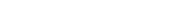This expression is described by the diagram above.

## Other formulas

As an expansion of the first law of thermodynamics
First law of thermodynamics
The first law of thermodynamics is an expression of the principle of conservation of work.The law states that energy can be transformed, i.e. changed from one form to another, but cannot be created nor destroyed...

, enthalpy can be related to several other thermodynamic formulas. As with the original definition of the first law;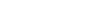where, as defined by the law;
dU represents the infinitesimal increase of the systematic
Thermodynamic system
A thermodynamic system is a precisely defined macroscopic region of the universe, often called a physical system, that is studied using the principles of thermodynamics....

or internal energy
δQ represents the infinitesimal amount of energy attributed or added to the system
δW represents the infinitesimal amount of energy acted out by the system on the surroundings.

As a differential expression, the value of H can be defined aswhere
δ represents the inexact differential
Inexact differential
An inexact differential or imperfect differential is a specific type of differential used in thermodynamics to express the path dependence of a particular differential. It is contrasted with the concept of the exact differential in calculus, which can be expressed as the gradient of another...

U is the internal energy
Internal energy
In thermodynamics, the internal energy is the total energy contained by a thermodynamic system. It is the energy needed to create the system, but excludes the energy to displace the system's surroundings, any energy associated with a move as a whole, or due to external force fields. Internal...

δQ = TdS is the energy added by heating during a reversible process
Reversible process (thermodynamics)
In thermodynamics, a reversible process, or reversible cycle if the process is cyclic, is a process that can be "reversed" by means of infinitesimal changes in some property of the system without loss or dissipation of energy. Due to these infinitesimal changes, the system is in thermodynamic...

δW = pdV is the work done by the system in a reversible process
dS is the increase in entropy
Entropy
Entropy is a thermodynamic property that can be used to determine the energy available for useful work in a thermodynamic process, such as in energy conversion devices, engines, or machines. Such devices can only be driven by convertible energy, and have a theoretical maximum efficiency when...

(joules per kelvin
Kelvin
The kelvin is a unit of measurement for temperature. It is one of the seven base units in the International System of Units and is assigned the unit symbol K. The Kelvin scale is an absolute, thermodynamic temperature scale using as its null point absolute zero, the temperature at which all...

)
p is the constant pressure
dV is an infinitesimal
Infinitesimal
Infinitesimals have been used to express the idea of objects so small that there is no way to see them or to measure them. The word infinitesimal comes from a 17th century Modern Latin coinage infinitesimus, which originally referred to the "infinite-th" item in a series.In common speech, an...

volume
T is the temperature (kelvin).

For a process that is not reversible, the above equation expressing dH in terms of dS and dp still holds because H is a thermodynamic state variable that can be uniquely specified by S and p. We thus have in general:
dH = TdS + Vdp

It is seen that, if a thermodynamic process is isobaric
Isobaric
Isobaric may refer to:*in thermodynamics, an isobaric process, i.e. one that is carried out at constant pressure;...

(i.e., occurs at constant pressure), then dp is zero and thus
dH = TdS ≥ δQ

The difference in enthalpy is the maximum thermal energy attainable from the system in an isobaric process
Isobaric process
An isobaric process is a thermodynamic process in which the pressure stays constant. The term derives from the Greek isos, , and barus,...

. This explains why it is sometimes called the heat content. That is, the integral of dH over any isobar in state space is the maximum thermal energy attainable from the system.

In a more general form, the first law describes the internal energy with additional terms involving the chemical potential
Chemical potential
Chemical potential, symbolized by μ, is a measure first described by the American engineer, chemist and mathematical physicist Josiah Willard Gibbs. It is the potential that a substance has to produce in order to alter a system...

and the number of particles of various types. The differential statement for dH then becomes: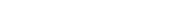where μi is the chemical potential for an i-type particle, and Ni is the number of such particles. It is seen that, not only must the Vdp term be set to zero by requiring the pressures of the initial and final states to be the same, but the μidNi terms must be zero as well, by requiring that the particle numbers remain unchanged. Any further generalization will add even more terms whose extensive differential term must be set to zero in order for the interpretation of the enthalpy to hold.

• Standard enthalpy change of formation (data table)
Standard enthalpy change of formation (data table)
These tables include heat of formation data gathered from a variety of sources, including the primary and secondary literature, as well as the NIST Chemistry WebBook...

• Calorimetry
Calorimetry
Calorimetry is the science of measuring the heat of chemical reactions or physical changes. Calorimetry is performed with a calorimeter. The word calorimetry is derived from the Latin word calor, meaning heat...

• Calorimeter
Calorimeter
A calorimeter is a device used for calorimetry, the science of measuring the heat of chemical reactions or physical changes as well as heat capacity. Differential scanning calorimeters, isothermal microcalorimeters, titration calorimeters and accelerated rate calorimeters are among the most common...

• Departure function
Departure function
In thermodynamics, a departure function is defined for any thermodynamic property as the difference between the property as computed for an ideal gas and the property of the species as it exists in the real world, for a specified temperature T and pressure P...

• Entropy
Entropy
Entropy is a thermodynamic property that can be used to determine the energy available for useful work in a thermodynamic process, such as in energy conversion devices, engines, or machines. Such devices can only be driven by convertible energy, and have a theoretical maximum efficiency when...

• Gibbs free energy
Gibbs free energy
In thermodynamics, the Gibbs free energy is a thermodynamic potential that measures the "useful" or process-initiating work obtainable from a thermodynamic system at a constant temperature and pressure...

• Hess's law
Hess's law
Hess' law is a relationship in physical chemistry named for Germain Hess, a Swiss-born Russian chemist and physician.The law states that the enthalpy change for a reaction that is carried out in a series of steps is equal to the sum of the enthalpy changes for the individual steps.The law is an...

• Isenthalpic process
Isenthalpic process
An isenthalpic process or isoenthalpic process is a process that proceeds without any change in enthalpy, H; or specific enthalpy, h....

• Stagnation enthalpy
• Thermodynamic databases for pure substances
Thermodynamic databases for pure substances
Thermodynamic databases contain information about thermodynamic properties for substances, the most important being enthalpy, entropy, and Gibbs free energy. Numerical values of these thermodynamic properties are collected as tables or are calculated from thermodynamic datafiles...